In a simultaneous throw of pair of dice, find the probability of getting:
Question:

In a simultaneous throw of pair of dice, find the probability of getting:

(i) 8 as the sum

(ii) a doublet

(iii) a doublet of prime numbers

(iv) a doublet of odd numbers

(v) a sum greater than 9

(vi) an even number on first

(vii) an even number on one and a multiple of 3 on the other

(viii) neither 9 nor 11 as the sum of the numbers on the faces

(ix) a sum less than 6

(x) a sum less than 7

(xi) a sum more than 7

(xii) at least once

(xiii) a number other than 5 on any dice.

(xiv) even number on each die                             [CBSE 2014, 2015]

(xv) 5 as the sum                                                  [CBSE 2014, 2015]

(xvi) 2 will come up at least once                        [CBSE 2015]

(xvii) 2 will not come either time                         [CBSE 2015]

Solution:

GIVEN: A pair of dice is thrown

TO FIND: Probability of the following:

Let us first write the all possible events that can occur

(1,1), (1,2), (1,3), (1,4), (1,5), (1,6),

(2,1), (2,2), (2,3), (2,4), (2,5), (2,6),

(3,1), (3,2), (3,3), (3,4), (3,5), (3,6),

(4,1), (4,2), (4,3), (4,4), (4,5), (4,6),

(5,1), (5,2), (5,3), (5,4), (5,5), (5,6),

(6,1), (6,2), (6,3), (6,4), (6,5), (6,6),

Hence total number of events is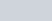(i) Favourable events i.e. getting the sum of numbers on the dice equal to 8

(2,6), (3,5), (4,4), (5,3), (6,2)

Hence total number of favourable events i.e. the sum of numbers on the dice equal to 8 is 5

We know that PROBABILITY =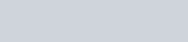Hence probability of getting the sum of numbers on the dice equal to 8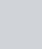(ii) Favourable events i.e. getting the same number on both the dice

(1,1), (2,2), (3,3) (4,4), (5,5), (6,6)

Hence total number of favourable events i.e. the same number on both the dice is 6

We know that PROBABILITY =Hence probability of getting the same number on both the dice=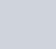(iii) Favourable events i.e. getting the same prime number on both the dice are

(2, 2), (3, 3) and (5, 5)

Hence total number of favourable events i.e. the same prime number on both the dice is 3

We know that PROBABILITY =Hence probability of getting the same prime number on both the dice=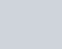(iv) Favourable events i.e. getting the same odd number on both the dice are

(1, 1), (3, 3) and (5, 5)

Hence total number of favourable events i.e. the same odd number on both the dice is 3

We know that PROBABILITY =Hence probability of getting the same odd number on both the dice is(v) Favourable events i.e. getting the total of numbers on the dice is greater than 9

(5,5), (5,6), (6,4), (4,6), (6,5), (6,6)

Hence total number of favourable events i.e. getting the total of numbers on the dice is greater than 9 is 6

We know that PROBABILITY =Hence probability of getting the total of numbers on the dice greater than 9 is(vi) Favourable events i.e. getting an even number on the first dice

(2,1), (2,2), (2,3), (2,4), (2,5), (2,6),

(4,1), (4,2), (4,3), (4,4), (4,5), (4,6),

(6,1), (6,2), (6,3), (6,4), (6,5), (6,6),

Hence total number of favourable events i.e. getting an even number on the first dice=18

We know that PROBABILITY =Hence probability of getting the an even number on the first dice=(vii) Favourable event that an even number on first dice and multiple of 3 on the other dice are (2,3), (2,6),(4,3), (4,6),(6,3), (6,6), (3,2), (6,2), (3,4), (6,4) and (3,6)

Hence total number of favourable events i.e. getting that an even number on first dice and multiple of 3 on the other dice is 11

We know that PROBABILITY =Hence probability of getting that an even number on one dice and multiple of 3 on the other dice is equal to(viii) Favourable event neither 9 nor 11 as the sum of the number of faces are

(1,1), (1,2), (1,3), (1,4), (1,5), (1,6),

(2,1), (2,2), (2,3), (2,4), (2,5), (2,6),

(3,1), (3,2), (3,3), (3,4), (3,5),

(4,1), (4,2), (4,3), (4,4), (4,6),

(5,1), (5,2), (5,3), (5,5),

(6,1), (6,2),), (6,4), (6,6),

Hence total number of favourable events i.e. getting neither 9 nor 11 as the sum of the number of faces is 30

We know that PROBABILITY =Hence probability of getting neither 9 nor 11 as the sum of the number of faces is equal to(ix) Favourable event i.e. sum less than 6

(1,1), (1,2), (1,3), (1,4),

(2,1), (2,2), (2,3)

(3,1), (3,2), (4,1),

Hence total number of favourable events i.e. sum less than 6 is 10

We know that PROBABILITY =Hence probability of getting sum less than 6 is equal to(x) Favourable event a sum less than 7

(1,1), (1,2), (1,3), (1,4), (1,5),

(2,1), (2,2), (2,3), (2,4),

(3,1), (3,2), (3,3),

(4,1), (4,2), (5,1),

Hence total number of favourable events i.e. sum less than 7 is 15

We know that PROBABILITY =Hence probability of getting sum less than 7 is equal to(xi) Favourable event a sum less than 7

(2,6), (3,5), (3,6), (4,4), (4,5), (4,6), (5,3), (5,4), (5,5), (5,6),

(6,2), (6,3), (6,4), (6,5), (6,6),

Hence total number of favourable events i.e. sum more than 7 is 15

We know that PROBABILITY =Hence probability of getting sum more than is equal to(xii) Favourable event at least once

(1,1), (1,2), (1,3), (1,4), (1,5), (1,6),(2,1), (3,1), (4,1), (5,1), (6,1),

Hence total number of favourable events i.e. at least once is 11

We know that PROBABILITY =Hence probability of getting at least once is equal to(xiii) Favourable event other than 5 on any dice

(1,1), (1,2), (1,3), (1,4), (1,6),

(2,1), (2,2), (2,3), (2,4), (2,6),

(3,1), (3,2), (3,3), (3,4), (3,6),

(4,1), (4,2), (4,3), (4,4), (4,6),

(6,1), (6,2), (6,3), (6,4), (6,6),

Hence total number of favourable events i.e. other than 5 on any dice is 25

We know that PROBABILITY =Hence probability of getting other than 5 on any dice is equal to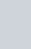(xiv) Favourable outcomes for getting even number on each die are

(2,2), (2,4), (2,6), (4,2), (4,4), (4,6), (6,2), (6,4), (6,6)

Hence, the number of favourable outcomes are 9.

$\therefore P($ even number on each die $)=\frac{\text { Favourable number of outcomes }}{\text { Total number of outcomes }}=\frac{9}{36}=\frac{1}{4}$

(xv) Favourable outcomes for getting 5 as the sum are

(1, 4), (2, 3), (3, 2), (4, 1)

Hence, the number of favourable outcomes are 4.

$\therefore \mathrm{P}($ getting 5 as the sum $)=\frac{\text { Favourable number of outcomes }}{\text { Total number of outcomes }}=\frac{4}{36}=\frac{1}{9}$

(xvi) Favourable outcomes for 2 coming up atleast once are

(1, 2), (2, 1), (2, 2), (2, 3), (2, 4), (2, 5), (2, 6), (3, 2), (4, 2), (5, 2), (6, 2)

Hence, the number of favourable outcomes are 11.

$\therefore \mathrm{P}(2$ will come up at least once $)=\frac{\text { Favourable number of outcomes }}{\text { Total number of outcomes }}=\frac{11}{36}$

(xvii) Favourable outcomes for 2 not coming either time are

(1, 1), (1, 3), (1, 4), (1, 5), (1, 6), (3, 1), (3, 3), (3, 4), (3, 5), (3, 6), (4, 1), (4, 3), (4, 4), (4, 5), (4, 6), (5, 1), (5, 3), (5, 4), (5, 5), (5, 6), (6, 1), (6, 3), (6, 4), (6, 5), (6, 6)

Hence, the number of favourable outcomes are 25.

$\therefore P\left(2\right.$ will not come up either time) $=\frac{\text { Favourable number of outcomes }}{\text { Total number of outcomes }}=\frac{25}{36}$